# Year 11 Revision - Level 3

This is a level 3 revision exercise to help prepare for Year 11/end of Key Stage 4 tests.Key stage:  KS 4

Curriculum topic:  Revision

Curriculum subtopic:  Year 11 Revision

Difficulty level:### QUESTION 1 of 10In this worksheet, you will find 40 questions that include many of the level 3 topics that you have learned this year.

Work through the questions carefully.

Calculators may not be used unless the question says that you may use one.

Good luck!

Is the following number rational or irrational?

5 - √12

rational

irrational

Write the following recurring decimal as a fraction in its lowest terms.Work out, without a calculator:

122/3 ×   182/3

Work out:

 7 - 5 ÷ 7 15 6 8

(Give your answer as a fraction, reduced to its lowest terms, e.g. 7/10.)You may use a calculator for this question.

Place these numbers in ascending order of size:

45.5%     5/11     4.5 × 10-1     0.1936½     (¾)3

## Column B

1 (smallest)
0.1936½
2
4.5 × 10-1
3
45.5%
4
¾3
5 (largest)
5/11You may use a calculator for this question.

Find the greatest and least possible areas in cm2 of the floor of a rectangular room with measurements:

268 cm by 322 cm

(Just write the number with no spaces between the numbers.)

 answers (cm2) least area greatest area

Simplify by rationalising the denominator of this fraction, giving your answer as a surd in the form a√b, where a and b are integers and b is as small as possible, or if the answer is a whole number, just state the whole number.(√20)/4

½√5

(√5)/4

Subtract:

2.5 × 10-5  -   6.35 × 10-4

-3.85 × 10-1

3.85 × 10-9

-6.1 × 10-4

Rearrange the following equation so that it is in the form ax + by = c,

where a ≥ 0, a, b and c are integers and the HCF of a, b, and c is 1.

4¾y = 4 - 2¼x

2x + 4y = 19

9x + 19y = 16

2¼x + 4¾y = 4

Solve the following equation for x:

 4x - 5 = 5x - 4 7 11

(Just write the number.)

18x2 - 87x + 14

(6x - 2) (3x - 7)

(3x - 2) (6x + 7)

(6x - 1) (3x - 14)

(2x - 7) (9x - 2)

17x + 21 = 3x2

x = 6.70

x = -4.04

x = 6.71

x = -1.04

Find all the x-intercepts of the graph with the following equation:

(i.e. where the line crosses the x-axis)

y = 8x2 - 10x - 3

(1½, 0)

(-1½, 0)

(-4, 0)

(-¼, 0)

Divide the following expression by 4x3.

40x7 + 36x6 - 16x4 - 20x3

10x4 + 9x3 - 4x - 5

36x5 + 9x2 - 4x - 5x

10x4 + 9x2 -12x - 5

Find the equation of the line perpendicular to 2x + 9y + 10 = 0, which passes through (1, 5).

2y - 9x - 1 = 0

2y + 9x + 1 = 0

9y - 2x = 1

Solve the following simultaneous equations:

18 - y2 = x2

y = 6 - x

 solutions x y

Choose the function which fits the following results:

f(-1) = -24.8

f(3/2) = (√5)3 - 25

f(2) = 0

f(x) = 5x - 25

f(x) = 5x + 5

f(x) = 25x - 5

Select the correct equation of the circle shown below:y2 + x2 = 4.5

y = x² = 4.5

x2 + y2 = 20.25

Simplify the following equation to one that is quadratic:

 2 + 1 = 7 2x - 1 4x + 3
56x² + 4x - 26 = 0

56x² - 24x - 10 = 0

56x² - 4x + 10 = 0You may use a calculator for this question.

Kate invests £65 000 at 8.5% compound interest p.a

Jack invests £65 000 at 8.5% simple interest p.a.

Neither of them touch their money for ten years.

How much more interest does Kate earn in pounds in ten years?

(Just write the number.)

3 painters take 2 days to paint a wall.

How many days would it take 4 painters to paint 2 similar walls?

(Just write the number.)You may use a calculator for this question.

The population of a certain species is expected to fall by x% each year.

After 10 years the population is halved.

Calculate x to 1 decimal place.You may use a calculator for this question.

Look at the function f(t) = 3t.

By what percentage does it change in the interval between t = 5 and t = 7?

(Just write the number.)

Look at this graph.

What is the equation of the blue line?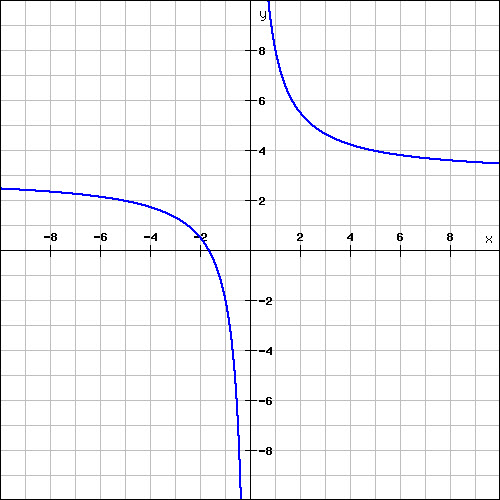y = 5/x + 3

y = 5/x - 3

x = 5/y + 3

x = 5/y - 3Calculate the total surface area (in cm2) of a cone with radius R equal to 3 cm and height H equal to 7 cm.

(3√58 + 9)π

3√58π

183π

(3√58 + 3)πYou may use a calculator for this question.

The diagram shows a 4 cm × 5 cm × 10 cm cuboid.

Calculate the perimeter of triangle ACG.

(Just write the number to 3 sig. figs.)You may use a calculator for this question.

The diagram below shows a Christmas tree with base at A and top at T.The distance AC is 28 metres.

The angle of elevation of T from C is 35°.

The angle of elevation of T from B is 12°.

Calculate the distance, BC in metres.You may use a calculator for this question.

In triangle PQR, which is not drawn to scale,

PQ = 12 cm, PR = 22 cm, and ∠QPR = 35º.

Calculate the length QR in cm to 3 sig. figs.

(Just write the number.)You may use a calculator for this question.

In triangle PQR, which is not drawn to scale,

PQ = 8 cm, PR = 11 cm and ∠PQR = 111°.

Use the sine rule to find ∠QPR to 1 dp.

(Just write the number.)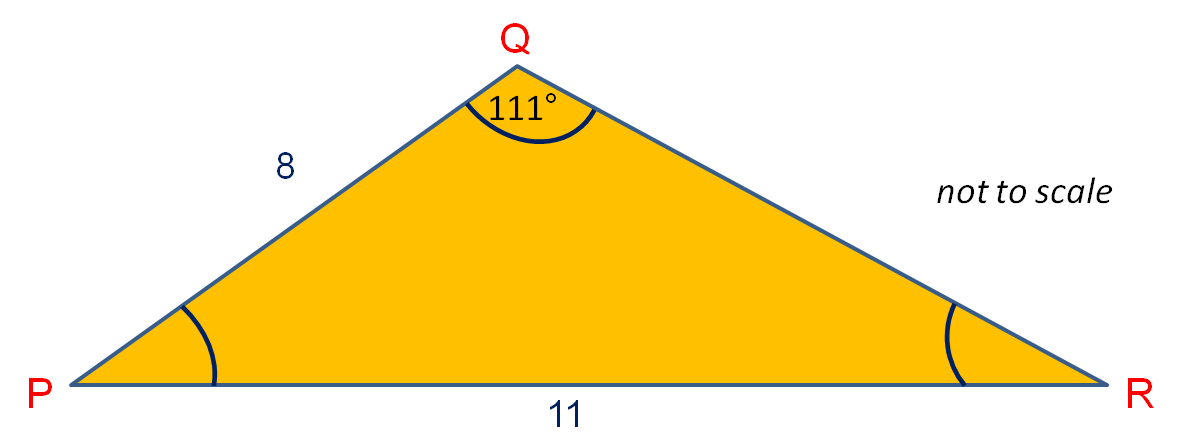In triangle PQR, which is not drawn to scale,

PQ = 6 cm, PR = 12 cm and ∠QRP = 30°.

Without the use of a calculator, find ∠QPR.

(Just write the number.)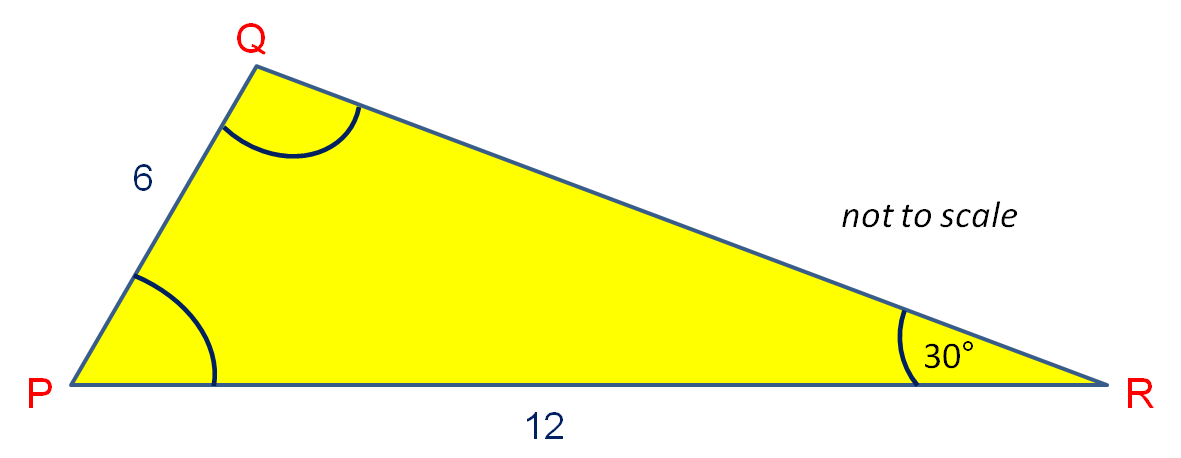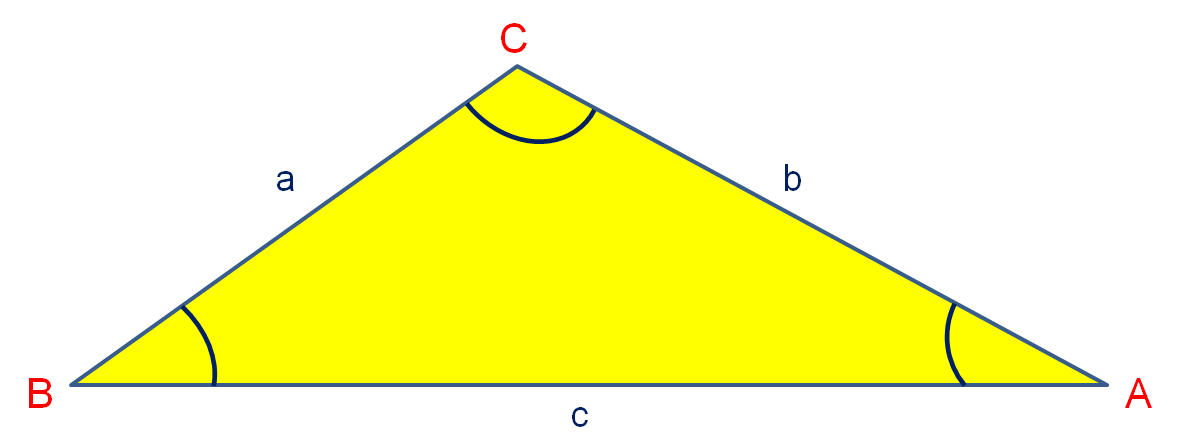In triangle ABC above,

a = 6.2 cm

b = 7 cm

∠BCA = 125º

Calculate the triangle's area in cm2 to 3 sig. figs.

(Just write the number.)

The diagram shows a circle, centre C and three points A, B and P on the circumference.

Calculate the angle ∠ABC.

(Just write the number.)A money bag contains 22 × £1 coins, 21 × 50p coins, 6 × 10p coins and 5 × 2p coins as well as a number of 20p coins.

The probability that a 20p coin is selected at random is 1/4.

Calculate the number of 20p coins in the bag.

Two fair tetrahedral dice are thrown. Each is numbered from 1 to 4.What is the probability that at least one die will show a 4?

(Write your answer as a fraction in the form a/b in its lowest terms.)

There are 16 red balls and 4 white balls in a bag.

Fatima randomly chooses 2 balls, removing each in turn from the bag.

What is the probability of choosing one of each colour?

A number between 380 and 440 is chosen at random.What is the probability that it is between 385 and 425?

(Write your answer as a fraction in the form a/b in its lowest terms.)

From the information in the box plot diagrams, match how each distribution is skewed or if it is symmetrical.## Column B

symmetrical
B
positively skewed
C
negatively skewed
A

Find the interquartile range of the following set of data:

12     4     17     12     10     18     2     31    13You may use a calculator for this question.

Over the course of five days, there were the following number of empty seats at a theatre.

12 seats, 14 seats, 10 seats, 20 seats, 24 seats

Calculate the standard deviation in the number of empty seats to 3 sig. figs.You may use a calculator for this question.

The following histogram shows the distribution of the lengths of adverts on one television channel during a particular day.

Work out how many TV adverts have been surveyed in total.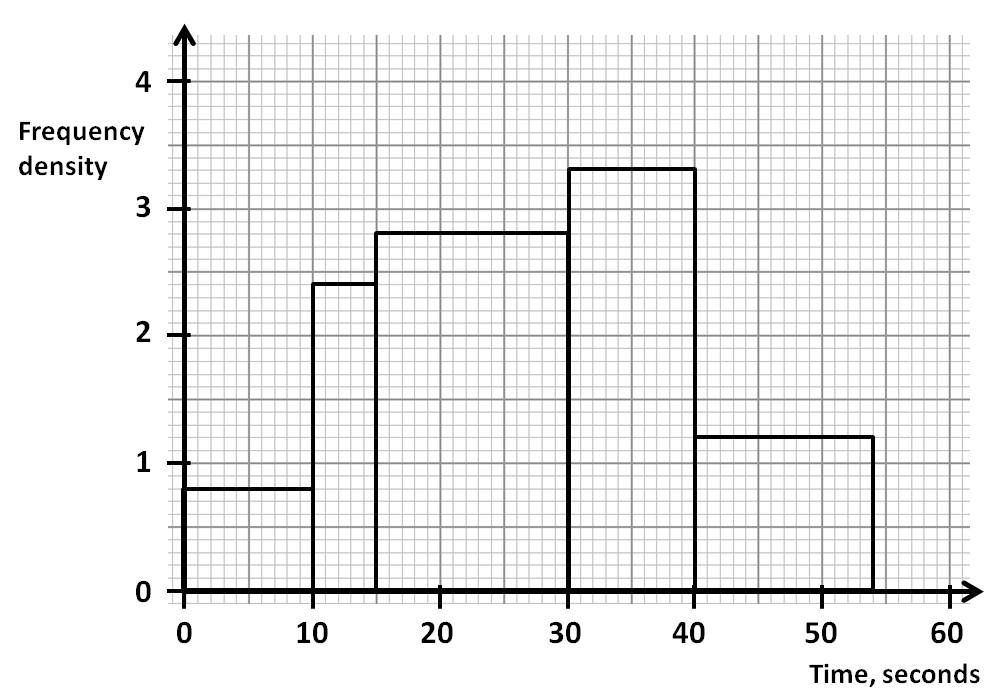• Question 1

Is the following number rational or irrational?

5 - √12

irrational
EDDIE SAYS
√12 is a non-recurring decimal.
• Question 2

Write the following recurring decimal as a fraction in its lowest terms.15/37
EDDIE SAYS
Three digits recur, so 405 over 999, which reduces.
Divide top and bottom by 27 or by 9 and 3 in stages.
• Question 3

Work out, without a calculator:

122/3 ×   182/3

36
EDDIE SAYS
(12 × 18)1/3
= 2161/3
= 3√216
= 6
6² = 36
• Question 4

Work out:

 7 - 5 ÷ 7 15 6 8

(Give your answer as a fraction, reduced to its lowest terms, e.g. 7/10.)

-17/35
EDDIE SAYS
First work out 5/6 ÷ 7/8 = 5/6 × 8/7 = 20/21.
Then work out 7/15 - 20/21 = 49/105 - 100/105 = -51/105 = -17/35.
• Question 5You may use a calculator for this question.

Place these numbers in ascending order of size:

45.5%     5/11     4.5 × 10-1     0.1936½     (¾)3

## Column B

1 (smallest)
¾3
2
0.1936½
3
4.5 × 10-1
4
5/11
5 (largest)
45.5%
EDDIE SAYS
0.753 = 0.421875
0.1936½ = √0.1936 = 0.44
4.5 × 10-1 = 0.45
5/11 = 0.4545...
45.5% = 0.455
• Question 6You may use a calculator for this question.

Find the greatest and least possible areas in cm2 of the floor of a rectangular room with measurements:

268 cm by 322 cm

(Just write the number with no spaces between the numbers.)

 answers (cm2) least area greatest area
EDDIE SAYS
Smallest possible dimensions are 267.5 by 321.5.
Largest possible dimensions are 268.5 by 322.5.
• Question 7

Simplify by rationalising the denominator of this fraction, giving your answer as a surd in the form a√b, where a and b are integers and b is as small as possible, or if the answer is a whole number, just state the whole number.(√20)/4
EDDIE SAYS
4/80 reduces to 1/20.
Multiply top and bottom by √20.
Remember 20 = 4 × 5.
• Question 8

Subtract:

2.5 × 10-5  -   6.35 × 10-4

-6.1 × 10-4
EDDIE SAYS
2.5 × 10-5 = 0.000025
6.35 × 10-4 = 0.000635
0.000025 - 0.000635 = -0.00061 = -6.1 × 10-4
• Question 9

Rearrange the following equation so that it is in the form ax + by = c,

where a ≥ 0, a, b and c are integers and the HCF of a, b, and c is 1.

4¾y = 4 - 2¼x

9x + 19y = 16
EDDIE SAYS
4¾y = 4 - 2¼x
2¼x + 4¾y = 4
Multiply by 4 to get:
9x + 19y = 16
• Question 10

Solve the following equation for x:

 4x - 5 = 5x - 4 7 11

(Just write the number.)

3
EDDIE SAYS
Multiply each side by 77 to get:
11(4x - 5) = 7(5x - 4)
44x - 55 = 35x - 28
9x = 27
x = 3
• Question 11

18x2 - 87x + 14

(6x - 1) (3x - 14)
EDDIE SAYS
We look for factor pairs that multiply to give 252 and add to give -87.
These are -84 and -3.
Factorise 18x² - 84x - 3x + 14 in pairs.
• Question 12

17x + 21 = 3x2

x = 6.71
x = -1.04
EDDIE SAYS
-3x2 + 17x + 21 = 0a=-3 b=17 c=21
• Question 13

Find all the x-intercepts of the graph with the following equation:

(i.e. where the line crosses the x-axis)

y = 8x2 - 10x - 3

(1½, 0)
(-¼, 0)
EDDIE SAYS
When y = 0,
8x² - 10x - 3 = 0
(2x - 3)(4x + 1) = 0
x = -¼, 1½
• Question 14

Divide the following expression by 4x3.

40x7 + 36x6 - 16x4 - 20x3

10x4 + 9x3 - 4x - 5
EDDIE SAYS
 40x7 + 36x6 - 16x4 - 20x3 4x3 4x3 4x3 4x3
• Question 15

Find the equation of the line perpendicular to 2x + 9y + 10 = 0, which passes through (1, 5).

2y - 9x - 1 = 0
EDDIE SAYS
Rearrange 2x + 9y + 10 = 0 to read 9y = -2x - 10 and then y = -2/9x - 10/9.
This line has a gradient of -2/9, so a perpendicular line will have gradient 9/2, i.e. y = 9/2x + c.
Put in the coordinates (1, 5) to get 5 = 9/2 + c, which gives c = 1/2.
Equation of perpendicular line is y =9/2x + 1/2 or 2y = 9x + 1.
• Question 16

Solve the following simultaneous equations:

18 - y2 = x2

y = 6 - x

 solutions x y
EDDIE SAYS
Substitute for y to get:
18 - (6 - x)2 = x2
18 - (36 - 12x + x2) = x2
-18 + 12x - x2 = x2
2x2 - 12x + 18 = 0
x2 - 6x + 9 = 0
(x - 3)2 = 0
x = 3
y = 3
• Question 17

Choose the function which fits the following results:

f(-1) = -24.8

f(3/2) = (√5)3 - 25

f(2) = 0

f(x) = 5x - 25
EDDIE SAYS
5-1 - 25 = 0.2 - 25
53/2 - 25 = (√5)³ - 25
52 - 25 = 25 - 25
• Question 18

Select the correct equation of the circle shown below:x2 + y2 = 20.25
EDDIE SAYS
Equation is x2 + y2 = 4.52 = 20.25.
• Question 19

Simplify the following equation to one that is quadratic:

 2 + 1 = 7 2x - 1 4x + 3
56x² + 4x - 26 = 0
EDDIE SAYS
Multiply everything by (2x - 1) and by (4x + 3).
Cancel matching brackets, top and bottom.
2(4x + 3) + 1(2x - 1) = 7(2x - 1)(4x +3)
2(4x + 3) + 1(2x - 1) = 7(8x² + 2x - 3)
8x + 6 + 2x - 1 = 56x² + 14x - 21
0 = 56x² + 14x - 21 - 10x - 5
0 = 56x² + 4x - 26
• Question 20You may use a calculator for this question.

Kate invests £65 000 at 8.5% compound interest p.a

Jack invests £65 000 at 8.5% simple interest p.a.

Neither of them touch their money for ten years.

How much more interest does Kate earn in pounds in ten years?

(Just write the number.)

26713.92
26 713.92
EDDIE SAYS
Kate's interest = 65 000 × 1.08510 - 65 000 = 81 963.92
Jack's interest = 65 000 × 0.085 × 10 = 55 250
81 963.92 - 55 250 = 26 713.92
• Question 21

3 painters take 2 days to paint a wall.

How many days would it take 4 painters to paint 2 similar walls?

(Just write the number.)

3
EDDIE SAYS
1 painter would take 6 days to paint a wall, so 12 days to paint 2 walls.
4 painters would take ¼ of 12 days.
• Question 22You may use a calculator for this question.

The population of a certain species is expected to fall by x% each year.

After 10 years the population is halved.

Calculate x to 1 decimal place.

6.7
EDDIE SAYS
(1 - x/100)10 = 0.5
Work out the 10th root of 0.5 = 0.933.
x/100 = 1 - 0.933 = 0.066967...
x = 6.6967
• Question 23You may use a calculator for this question.

Look at the function f(t) = 3t.

By what percentage does it change in the interval between t = 5 and t = 7?

(Just write the number.)

800
EDDIE SAYS
35 = 243
37 = 2187
Actual increase = 2187 - 243 = 1944
% increase = 1944 ÷ 243 × 100 = 800
• Question 24

Look at this graph.

What is the equation of the blue line?y = 5/x + 3
EDDIE SAYS
This is the exponential curve y = 5/x shifted up 3 units.
Test out different values, e.g. when x = 5, y = 1 + 3 = 4.
• Question 25Calculate the total surface area (in cm2) of a cone with radius R equal to 3 cm and height H equal to 7 cm.

(3√58 + 9)π
EDDIE SAYS
Slant length = √(3² + 7²) = √58
Curved Surface Area = π × 3 × √58
Base Area = π × 3² = 9π
Total Area = 3√58π + 9π = (3√58 + 9)π
• Question 26You may use a calculator for this question.

The diagram shows a 4 cm × 5 cm × 10 cm cuboid.

Calculate the perimeter of triangle ACG.

(Just write the number to 3 sig. figs.)27.6
EDDIE SAYS
Use Pythagoras' Theorem to get:
AC² = 4² + 10² = 16 + 100 = 116
AC = √116

AG² = 4² + 5² + 10² = 16 + 25 + 100 = 141
AG = √141

Perimeter = √116 + √141 + 5 = 27.64467...
• Question 27You may use a calculator for this question.

The diagram below shows a Christmas tree with base at A and top at T.The distance AC is 28 metres.

The angle of elevation of T from C is 35°.

The angle of elevation of T from B is 12°.

Calculate the distance, BC in metres.

64.2
EDDIE SAYS
tan35° = AT/AC
AT = 28tan35° = 19.6058...
AB = AT/tan12° = 19.6058 ÷ tan12° = 92.238...
BC = AB - AC = 92.238 - 28 = 64.238...
• Question 28You may use a calculator for this question.

In triangle PQR, which is not drawn to scale,

PQ = 12 cm, PR = 22 cm, and ∠QPR = 35º.

Calculate the length QR in cm to 3 sig. figs.

(Just write the number.)14.0
EDDIE SAYS
Use the cosine rule to get QR² = 12² + 22² - 2 × 12 × 22 × cos 35°.
• Question 29You may use a calculator for this question.

In triangle PQR, which is not drawn to scale,

PQ = 8 cm, PR = 11 cm and ∠PQR = 111°.

Use the sine rule to find ∠QPR to 1 dp.

(Just write the number.)26.2
EDDIE SAYS
 11 = 8 sin 111° sin ∠QRP

∠QPR = 180° - 111° - ∠QRP
• Question 30

In triangle PQR, which is not drawn to scale,

PQ = 6 cm, PR = 12 cm and ∠QRP = 30°.

Without the use of a calculator, find ∠QPR.

(Just write the number.)60
EDDIE SAYS
 6 = 12 sin 30° sin ∠PQR

You should know that sin 30° = 0.5.
So sin ∠PQR = 1, which means that ∠PQR = 90°.
This is a right-angled triangle.
• Question 31In triangle ABC above,

a = 6.2 cm

b = 7 cm

∠BCA = 125º

Calculate the triangle's area in cm2 to 3 sig. figs.

(Just write the number.)

17.8
EDDIE SAYS
Area = ½ × 6.2 × 7 × sin125°
• Question 32

The diagram shows a circle, centre C and three points A, B and P on the circumference.

Calculate the angle ∠ABC.

(Just write the number.)53
EDDIE SAYS
∠ACB = 2∠APB so a = 74°
Triangle ACB is isosceles.
∠ABC = (180° - 74°) ÷ 2 = 106° ÷ 2 = 53°
• Question 33

A money bag contains 22 × £1 coins, 21 × 50p coins, 6 × 10p coins and 5 × 2p coins as well as a number of 20p coins.

The probability that a 20p coin is selected at random is 1/4.

Calculate the number of 20p coins in the bag.

18
EDDIE SAYS
Let n be the number of 20p coins.
n/(22 + 21 + 6 + 5 + n) = 1/4
n/(54 + n) = 1/4
Multiply both sides by 4 and by (54 + n) to get:
4n = (54 + n)
3n = 54
n = 18
• Question 34

Two fair tetrahedral dice are thrown. Each is numbered from 1 to 4.What is the probability that at least one die will show a 4?

(Write your answer as a fraction in the form a/b in its lowest terms.)

7/16
EDDIE SAYS
List out all 16 possibilities.
7 of these have at least one 4.
• Question 35

There are 16 red balls and 4 white balls in a bag.

Fatima randomly chooses 2 balls, removing each in turn from the bag.

What is the probability of choosing one of each colour?

32/95
EDDIE SAYS
16/20 × 4/19 + 4/20 × 16/19
• Question 36

A number between 380 and 440 is chosen at random.What is the probability that it is between 385 and 425?

(Write your answer as a fraction in the form a/b in its lowest terms.)

2/3
EDDIE SAYS
440 - 380 = 60
425 - 385 = 40
Probability = 40/60 = 2/3
• Question 37

From the information in the box plot diagrams, match how each distribution is skewed or if it is symmetrical.## Column B

symmetrical
B
positively skewed
A
negatively skewed
C
EDDIE SAYS
In A, the median is nearer to the lower quartile, so it is positively skewed.
In B, the median is in the middle, so it is symmetrical.
In C, the median is nearer to the upper quartile, so it is negatively skewed.
• Question 38

Find the interquartile range of the following set of data:

12     4     17     12     10     18     2     31    13

10.5
EDDIE SAYS
First place the numbers in order to get:
2, 4, 10, 12, 12, 13, 17, 18, 31

Upper Quartile is the Median of 13, 17, 18, 31, which is 17.5.
Lower Quartile is the Median of 2, 4, 10, 12, which is 7.
Interquartile Range is 17.5 - 7 = 10.5.
• Question 39You may use a calculator for this question.

Over the course of five days, there were the following number of empty seats at a theatre.

12 seats, 14 seats, 10 seats, 20 seats, 24 seats

Calculate the standard deviation in the number of empty seats to 3 sig. figs.

5.22
EDDIE SAYS

Step 1

Mean = (12 + 14 + 10 + 20 + 24) ÷ 5 = 80 ÷ 5 = 16

Step 2

(12 - 16)2 = (-4)2 = 16

(14 - 16)2 = (-2)2 = 4

(10 - 16)2 = (-6)2 = 36

(20 - 16)2 = (4)2 = 16

(24 - 16)2 = (8)2 = 64

Step 3

Mean of 16, 4, 36, 16 and 64 = (16 + 4 + 36 + 16 + 64) ÷ 5 = 136 ÷ 5 = 27.2

Variance = 27.2
Standard Deviation = √27.2 = 5.22
• Question 40You may use a calculator for this question.

The following histogram shows the distribution of the lengths of adverts on one television channel during a particular day.

Work out how many TV adverts have been surveyed in total.113
EDDIE SAYS
We add up all the frequencies to get:
(0.8 × 10) + (2.4 × 5) + (2.8 × 15) + (3.3 × 10) + (1.2 × 15)
= 8 + 12 + 42 + 33 + 18 = 113
---- OR ----

Sign up for a £1 trial so you can track and measure your child's progress on this activity.

### What is EdPlace?

We're your National Curriculum aligned online education content provider helping each child succeed in English, maths and science from year 1 to GCSE. With an EdPlace account you’ll be able to track and measure progress, helping each child achieve their best. We build confidence and attainment by personalising each child’s learning at a level that suits them.